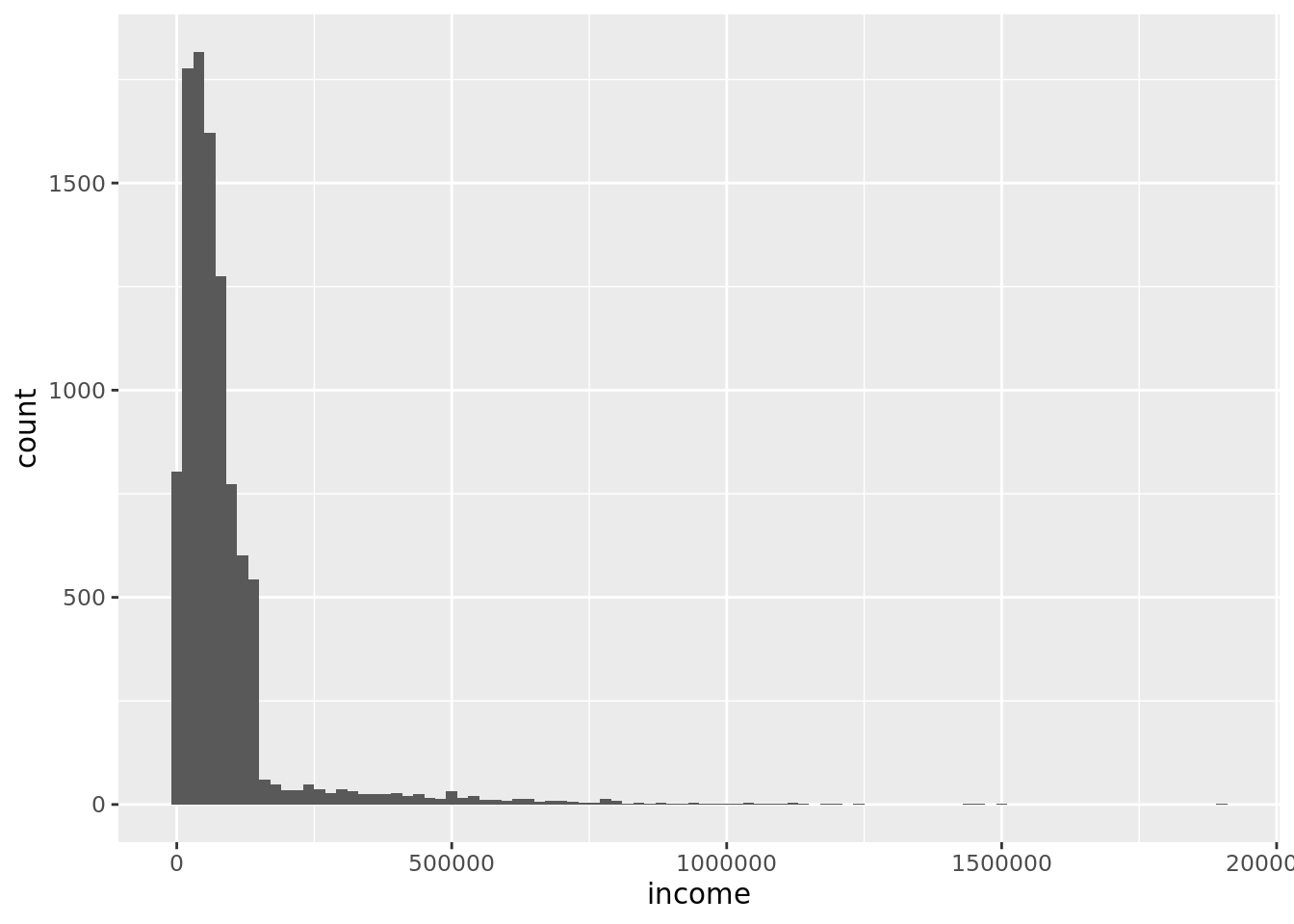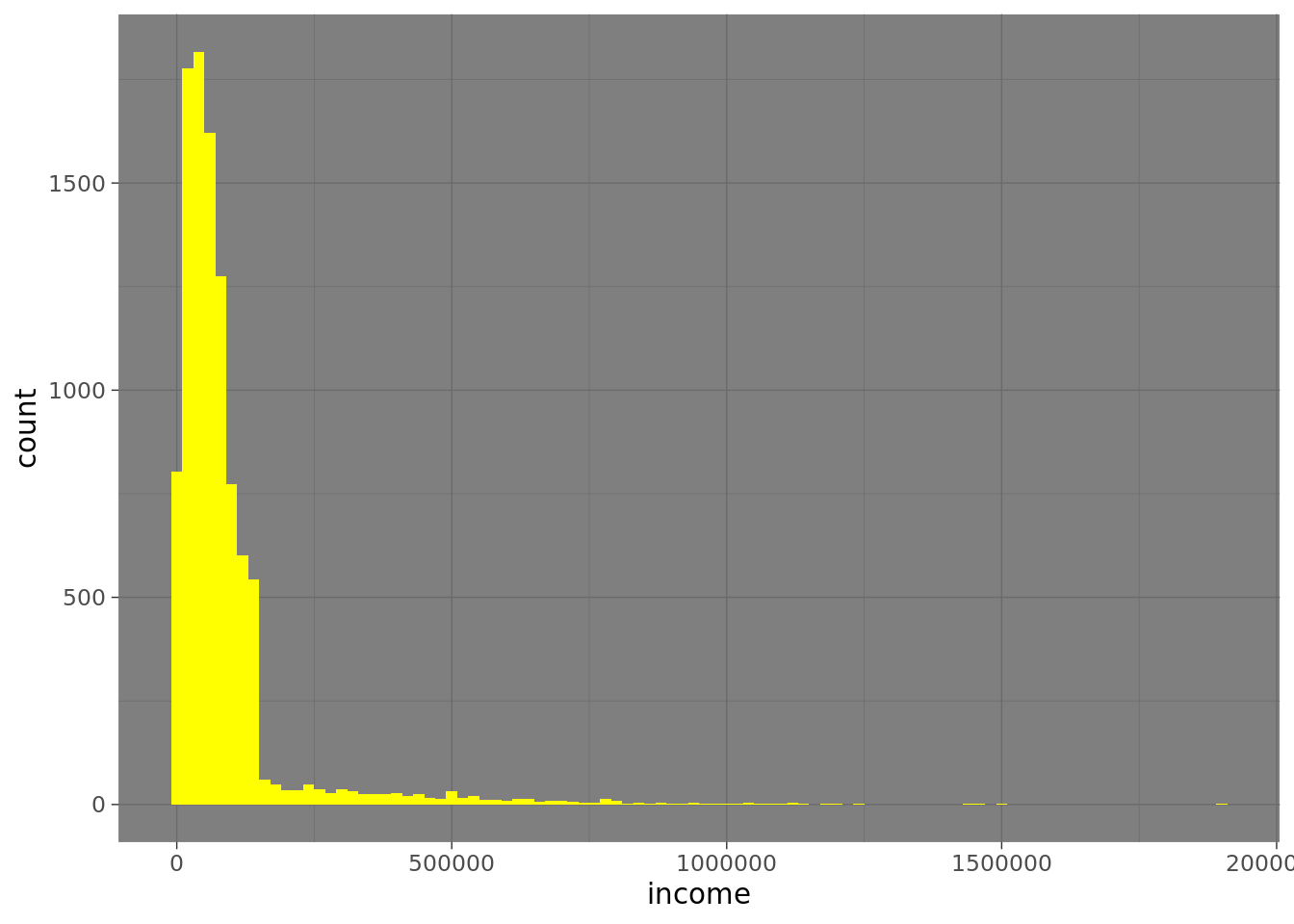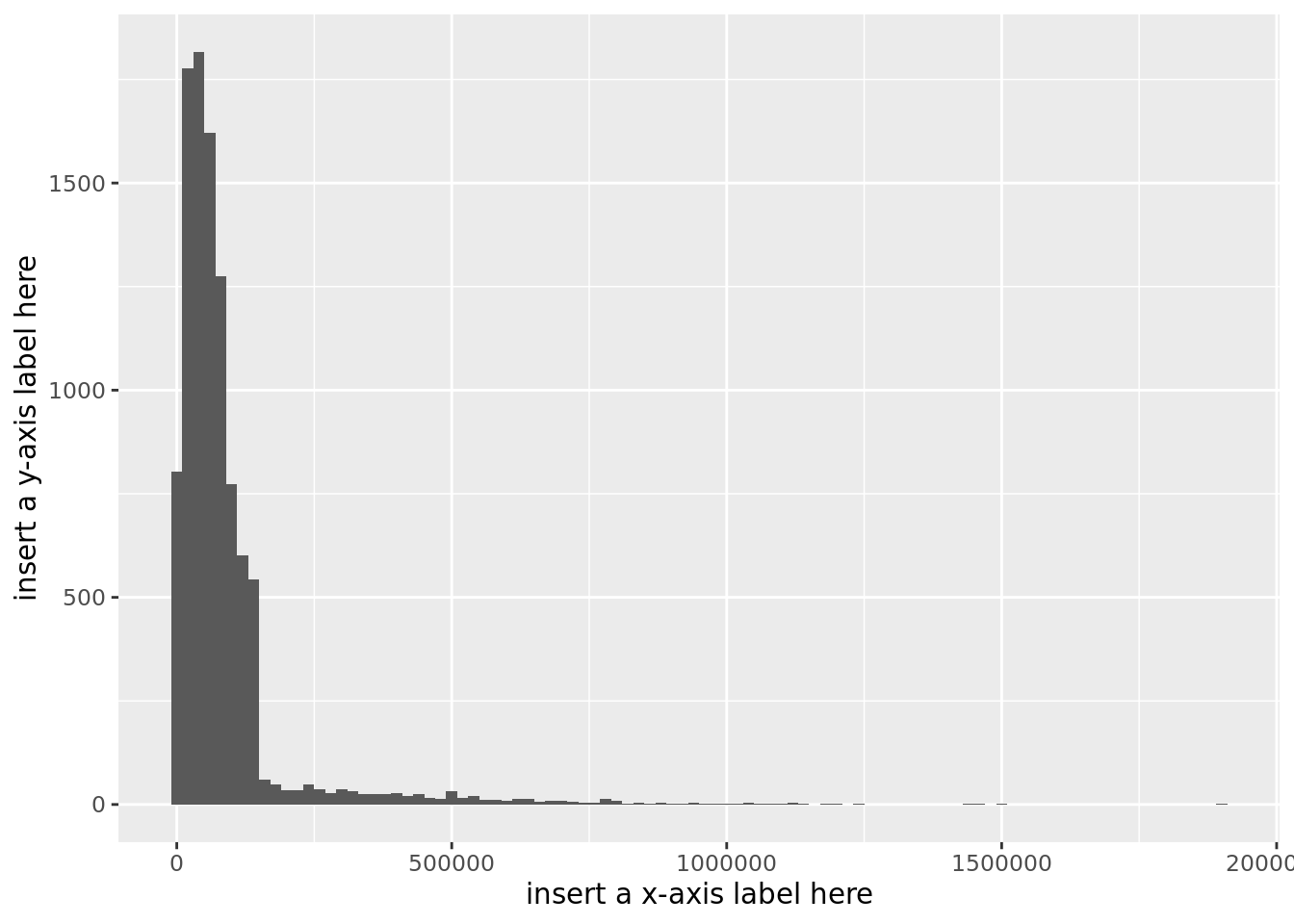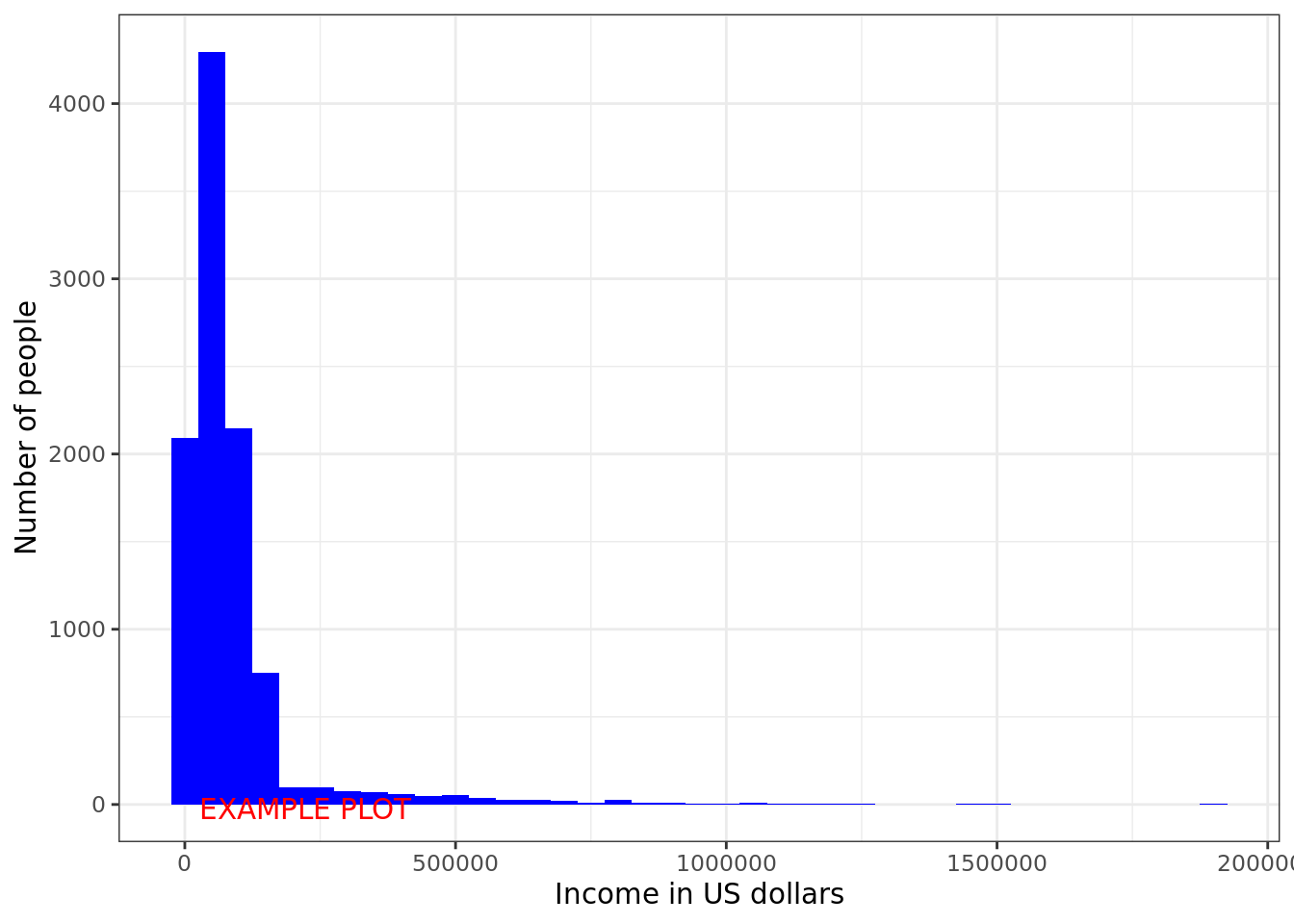## Before you start…

Before starting this exercise, you should have had a brief introduction to getting and using RStudio. If not, take a look at the Introduction to RStudio.

## How to use these worksheets

Throughout this worksheet, you’ll see the commands you should type into RStudio inside a grey box, followed by the output you should expect to see in one or more white boxes. Any differences in the colour of the text can be ignored.

Each command in this worksheet is followed by one or more explanation sections - those are there to help you understand how the commands work and how to read the output they produce.

First, we need to load a package called tidyverse. A package is an extension to R that adds new commands. Nearly everything we’ll do in this course uses the tidyverse package, so pretty much every project starts with this instruction.

Here’s how you do this:

1. Type (or copy and paste) the command in the grey box below into the script you have already created on RStudio (top left window, exploring.R). Use line 2 of the script. (Line 1 contains the comment you entered in the last worksheet).

2. Save your script again (click the Save icon), so you don’t lose anything. Do this each time you add something important to your script.

3. Now ask RStudio to run this command. You do this by putting your cursor on the line of the script window containing the command, and pressing CTRL+ENTER (i.e. press the key marked ‘Ctrl’ and the RETURN or ENTER key together). The line is automatically copied to your Console window and run.

library(tidyverse)
── Attaching packages ─────────────────────────────────────── tidyverse 1.3.1 ──
✓ ggplot2 3.3.4     ✓ purrr   0.3.4
✓ tibble  3.1.2     ✓ dplyr   1.0.6
✓ tidyr   1.1.3     ✓ stringr 1.4.0
✓ readr   1.4.0     ✓ forcats 0.5.1
── Conflicts ────────────────────────────────────────── tidyverse_conflicts() ──
x dplyr::lag()    masks stats::lag()

When you do this, RStudio will print some text to the Console (shown in the white box, above). This text tells you that the tidyverse package has loaded (“attached”) some other packages (e.g. dplyr). It also tells you that the dplyr package changes the way some commands in R work (“conflicts”). That’s OK.

If you get an output that includes the word ‘error’, please see common errors.

Now, we’re going to load some data on the income of 10,000 people in the United States of America. I’ve made up this dataset for teaching purposes, but it’s somewhat similar to large open data sets available on the web, such as US Current Population Survey.

Copy the command in the grey box to line 3 of your script in RStudio and then press CTRL+ENTER to run it: (don’t forget to save your script)

cpsdata <- read_csv("http://www.willslab.org.uk/cps2.csv")
Parsed with column specification:
cols(
ID = col_double(),
sex = col_character(),
native = col_character(),
blind = col_character(),
hours = col_double(),
job = col_character(),
income = col_double(),
education = col_character()
)

### Explanation of command

There are three parts to the command cpsdata <- read_csv("http://www.willslab.org.uk/cps2.csv"):

1. The first part of the command is cpsdata. This gives a name to the data we are going to load. We’ll use this name to refer to it later, so it’s worth using a name that is both short and meaningful. I’ve called it cpsdata because it’s somewhat similar to data from the US Current Population Survey, but you can give data pretty much any name you choose (e.g. fart).

2. The bit in the middle, <-, is an arrow and is typed by pressing < and then -, without a space. This arrow means “put the thing on the right of the arrow into the thing on the left of the arrow”.

3. The last part of the command is read_csv("http://www.willslab.org.uk/cps2.csv"). It’s a way of downloading data from the Internet. The part inside the speech marks, http://www.willslab.org.uk/cps2.csv, is a web address, such as you’d use to access any other web page (e.g. http://www.twitter.com/ajwills72)

### Explanation of output

R likes to print things in red sometimes – this does not mean there’s a problem. If there’s a problem, it will actually say ‘error’. The output here tells us that R has loaded the data, which has eight parts (columns, or cols). It gives us the name of the columns (ID, sex, ...) and tells us what sort of data each column contains: character means the data is words (e.g. ‘female’), integer means the data is a whole number (e.g. ‘42’).

If you get an error here, please see common errors.

## Inspecting data

Next, we’ll take a peek at these data. You can do this by clicking on the data in the Environment tab of RStudio, see Introduction to RStudio.

We can now see the data set (also known as a data frame). We can see that this data frame has 8 columns and 10000 rows. Each row is one person, and each column provides some information about them. Below is a description of each of the columns. Where you see NA this means this piece of data is missing for this person – quite common in some real datasets.

Here’s what each of the columns in the data set contains:

Column Description Values
ID Unique anonymous participant number 1-10,000
sex Biological sex of participant male, female
native Participant born in the US? foreign, native
blind Participant blind? yes, no
hours Number of hours worked per week a number
job Type of job held by participant: charity, nopay, private, public
income Annual income in dollars a number
education Highest qualification obtained grade-school, high-school, bachelor, master, doctor

## Calculating a mean

Now we have these data, one question we can ask is “what is the average income of people in the U.S.?” (or, at least, in this sample). In this first example, we’re going to calculate the mean income.

I’m sure you learned about means in school but, as a reminder, you calculate a mean by adding up all the incomes and dividing by the number of incomes. Our sample has 10,000 participants, so this would be a long and tedious calculation – and we’d probably make an error.

It would also be a little bit tedious and error prone in a spreadsheet application (e.g. Excel, Libreoffice Calc). There are some very famous cases of these kinds of “Excel errors” in research, e.g. genetics, economics.

In R, we can calculate the mean instantly, and it’s harder to make the sorts of errors that are common in Excel-based analysis.

To calculate mean income in R, add the following command to line 4 of your script and run it:

cpsdata %>% summarise(mean(income))
# A tibble: 1 x 1
mean(income)
<dbl>
1         87293.

Your output will tell you the mean income in this sample – it’s the last number on the bottom right, and it’s approximately $87,000. If you’re happy with the output you’ve got, move on to the next section. If you would like a more detailed explanation of this output, see more on tibbles. If you get an error here, please see common errors. RECORD YOUR ANSWER - Type the exact mean income into the Answer Section of Psyc:EL. ### Explanation of command This command has three components: 1. The bit on the left, cpsdata, is our data frame, which we loaded and named earlier. 2. The bit in the middle, %>%, is called a pipe. Its job is to send data from one part of your command to another. It is typed by pressing % then > then %, without spaces. So cpsdata %>% sends our data frame to the next part of our command. 3. The bit on the right, summarise(mean(income)) is itself made up of parts. The command summarise does as the name might suggest, it summarises a set of data (cpsdata in this case) into a single number, e.g. a mean. The mean command indicates that the type of summary we want is a mean (there are also a number of other types of summary, as we’ll see later). Finally, income is the name of the column of cpsdata we want to take the mean of – in this case, the income of each individual. ## Calculating a median Now we’re going to calculate the median income of the people in this sample. As you learned in school, you calculate a median by putting all the numbers into rank order and then picking the number in the middle. As with the calculation of mean outcome, R allows us to calculate the median quickly and without error. This time, I haven’t given you the command you need to type – your task is to work out what you need to type. Re-read the explanation above for clues if you need them. The way to indicate that the summary you want is a median is to use the command median. If you’ve entered the correct command, you’ll get this answer: # A tibble: 1 x 1 median(income) <dbl> 1 56952. RECORD YOUR ANSWER - Type the exact command you used to calculate median income into the Answer Section of Psyc:EL. ## Dealing with missing data To calculate the mean number of hours worked per week, we have to deal with the fact that there is some missing data - we don’t know for all 10,000 people how many hours they work in a week, because they didn’t all tell us. To get a mean of those who did tell us, we tell R to ignore the missing data, like this: cpsdata %>% summarise(mean(hours, na.rm = TRUE)) # A tibble: 1 x 1 mean(hours, na.rm = TRUE) <dbl> 1 38.9 ### Explanation rm is short for ‘remove’, but ‘ignore’ would be a more accurate description, as this command doesn’t delete the NA entries in cpsdata, it just ignores them. So na.rm = TRUE means “ignore the missing data”. If you get an error here, please see common errors. ## Introduction to graphs In the last two exercises, we found that median US income was much lower than mean US income. To help us explore why that might be, we’re going to look at the distribution of incomes. Again, we’re going to use a concept you learned in school – we’re going to produce a histogram. A histogram is a graph that shows us how many people have an income that is within a number of equal, consecutive, ranges (also called bins). In this example, we’re going to count how many people earn$0-19,999, how many earn $20,000-39,999, and so on, until we reach the highest income in the sample. So, our bin width will be$20,000. We’re then going to represent these counts by the height of a series of bars.

In R, we can do this with a single command:

cpsdata %>% ggplot(aes(income)) + geom_histogram(binwidth=20000)### Explanation of command

1. The first part, cpsdata %>% works the same way as in the previous examples. It takes the data from cpsdata and pipes it to our graphing command ggplot.

2. The command ggplot needs to know which of the columns you want to show on your graph, and we use the command aes (short for “aesthetics”) to specify this. In this case, we want to plot the incomes, so the command is ggplot(aes(income))

3. We also have to tell R how we want to graph the income data. The command geom_histogram says that we want a histogram (geom_ in this context just means graph). We also need to specify the bin width for our histogram, using binwidth.

## Customising graphs

There are a lot of ways to modify the standard graphs produced by R, to make them look the way you want. Here, we’re going to try just a few of them.

### Changing the theme

We can change the overall look of a graph by changing the theme. In this example, we use a lighter background by adding the command + theme_light():

cpsdata %>% ggplot(aes(income)) + geom_histogram(binwidth=20000) + theme_light()

There are quite a lot of different themes. Try replacing light above with one of the following: bw, classic, gray, linedraw, light, minimal, void, dark

### Changing the colour

We can also change the way particular parts of the graph look – for example, changing the colour of the bars on the histogram, using the command fill. Here’s one particularly nasty-looking example:

cpsdata %>% ggplot(aes(income)) + geom_histogram(fill = 'yellow', binwidth=20000) + theme_dark()Try replacing yellow with some other colour name. R knows quite a lot of colour names.

### Changing the labels

We can also change the labels that appear on the x-axis and on the y-axis. Here’s an example with some not-very-helpful labels:

cpsdata %>% ggplot(aes(income)) + geom_histogram(binwidth=20000) +
xlab('insert a x-axis label here') + ylab('insert a y-axis label here')### Lab book exercise - Upload a graph

Generate a histogram of these data with a bin width of \$50,000. The histogram bars should be blue, and you should use theme_bw. Give your x-axis and y-axis meaningful labels. If you get it right, your graph should look something like this (without the words “EXAMPLE PLOT”, of course):Now, export your histogram, using the Export icon on RStudio’s Plots window, and selecting “Save as image…”. Give it a meaninful file name (e.g. “ExploringIncomes”) and click ‘Save’.

RECORD YOUR ANSWER - Psyc:EL and RStudio Online are entirely separate web pages that don’t talk to each other, so you’ll need to download your graph from RStudio Online and then upload it to Psyc:EL. Here’s how:

1. Open your computer’s Downloads folder (e.g. on a Windows 10 machine, click on the little yellow folder at the bottom of the screen, and then click on “Downloads”).